This document walk you through the different options that help you produce desired legend.

``````library(wheatmap)
a <- matrix(rnorm(100),nrow=10)
d <- both.cluster(a)``````

Two major function for producing legends are `WLegendH` (horizontal legend) and `WLegendV` (vertical legend). The following just placed a vertical legend to the bottom right of the dendrogram,

``````p <- WHeatmap(d\$mat,name='a') + WDendrogram(d\$column.clust, RightOf('a'), facing = 'left')
p + WLegendV('a',BottomRightOf())``````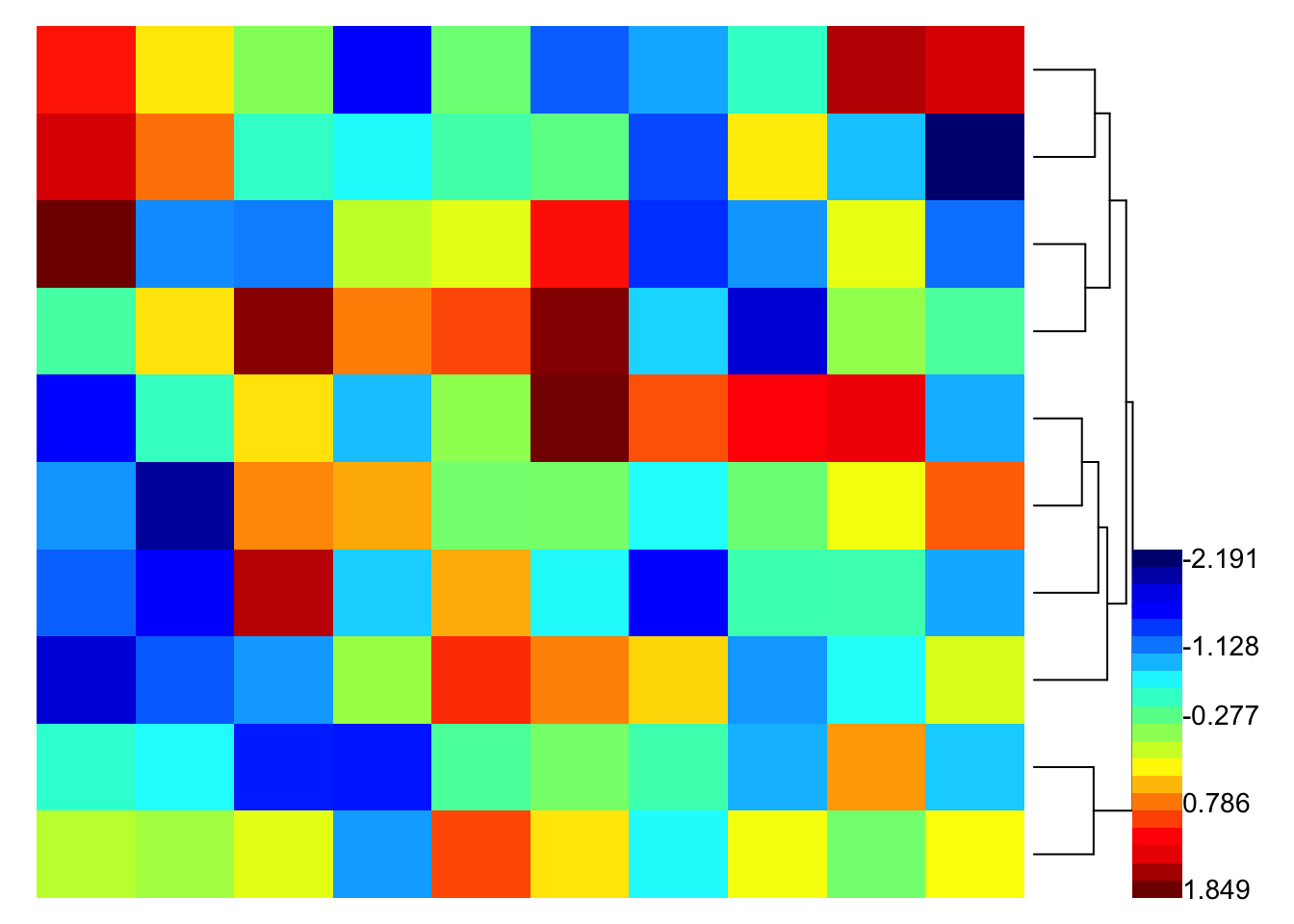We can use `h.pad` (short for “horizontal padding”) option in `BottomRightOf` to make some space between the dendrogram and the legend.

``p + WLegendV('a',BottomRightOf(h.pad=0.05))``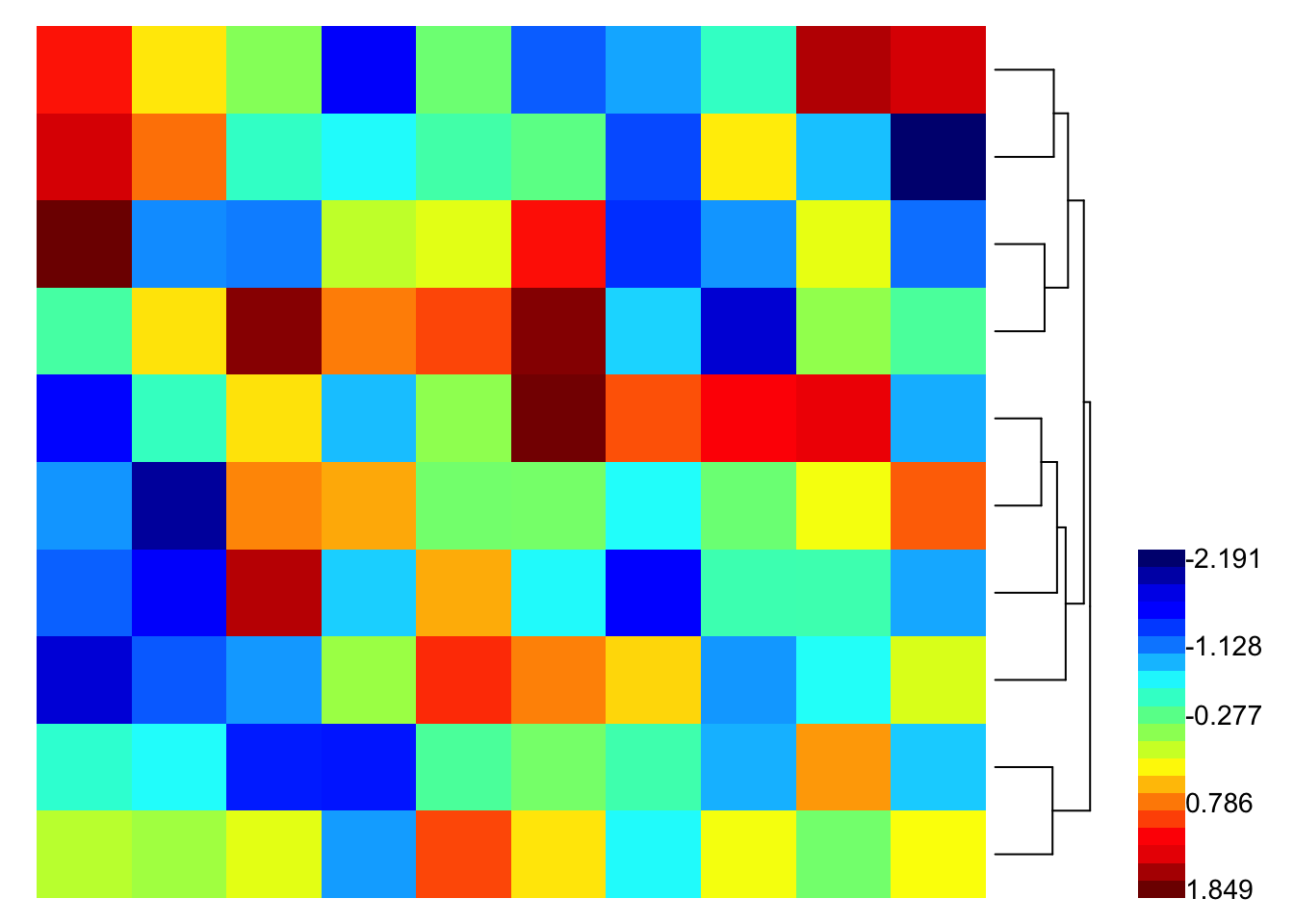The `height` option in `WLegendV` and `WLegendH`

``p + WLegendV('a',BottomRightOf(h.pad=0.05), height=0.04)``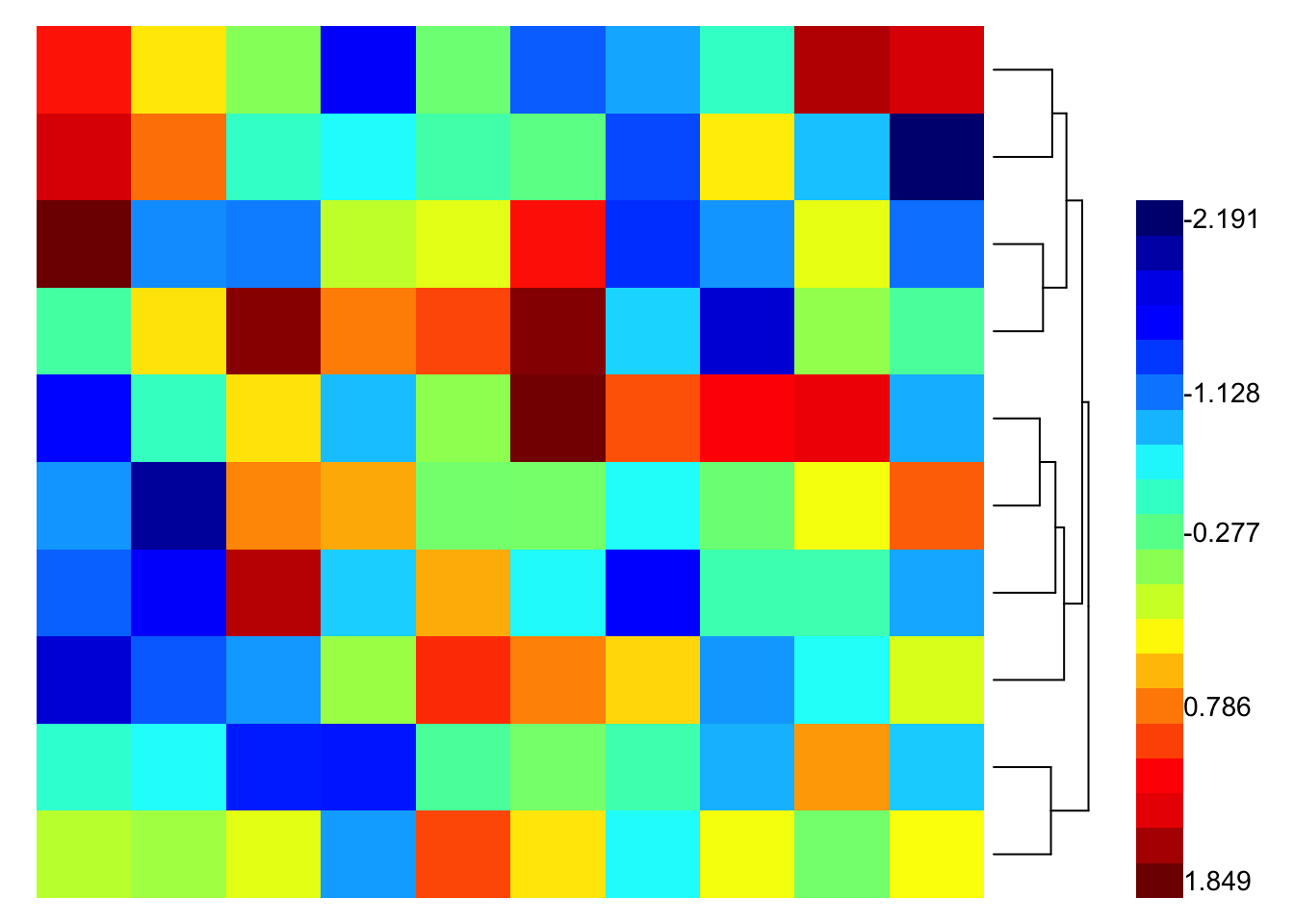# anchoring

The `just` option in `BottomRightOf` specify how the legend gets anchored to the bottom right of the dendrogram. The format is (horizontal anchor, vertical anchor). horizontal anchor can be c(‘left’,‘center’,‘right’) and vertical anchor can be c(‘bottom’,‘center’,‘top’). The default for `BottomRightOf` is c(‘left’,‘bottom’). Here we switch the legend anchor to c(‘left’,‘top’).

``p + WLegendV('a',BottomRightOf(h.pad=0.05, just=c('left','top')), height=0.02)``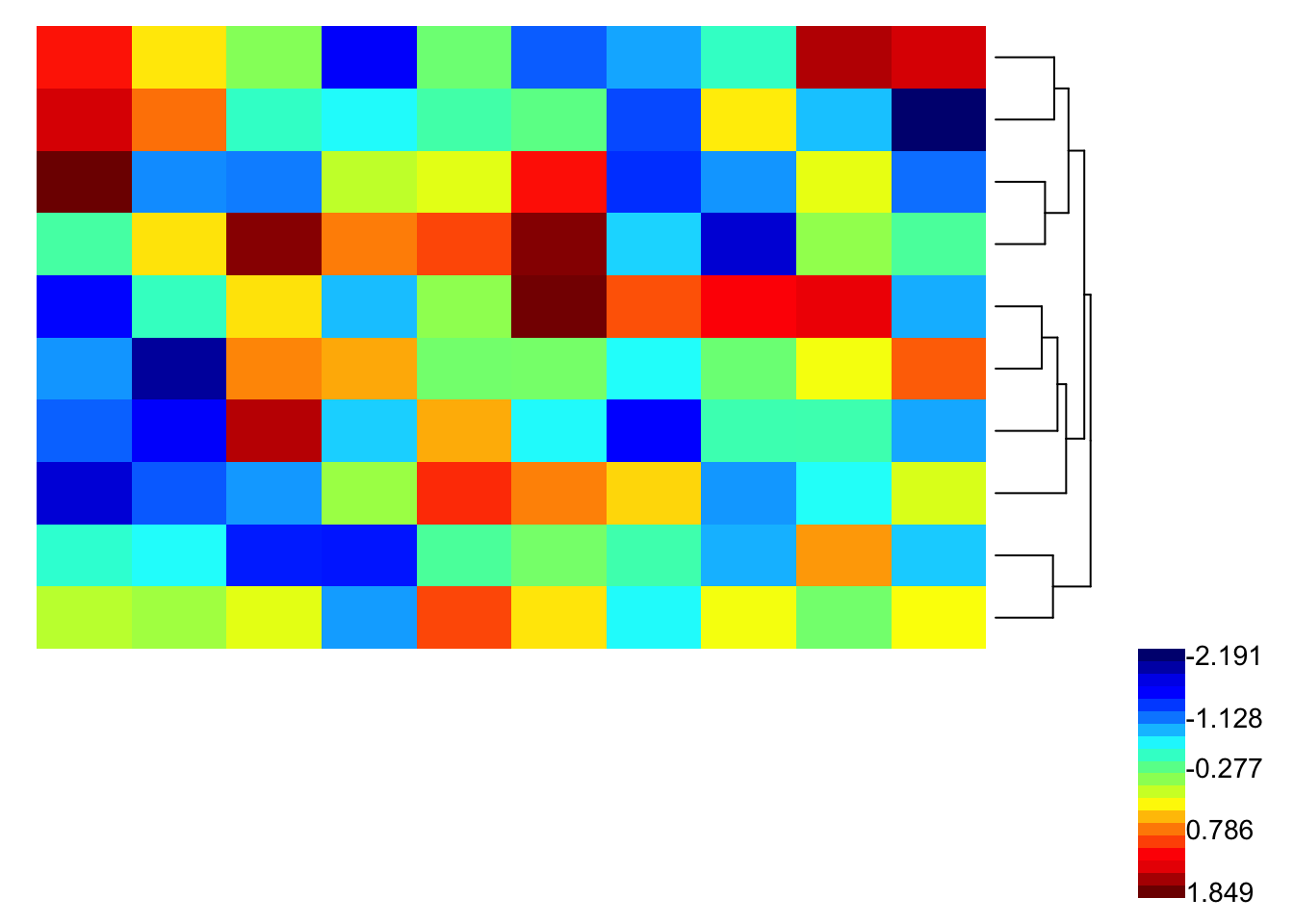So far we have covered vertical legend. Let’s look at horizontal legend.

``p + WLegendH('a',BottomRightOf(h.pad=0.05, just=c('left','top')))``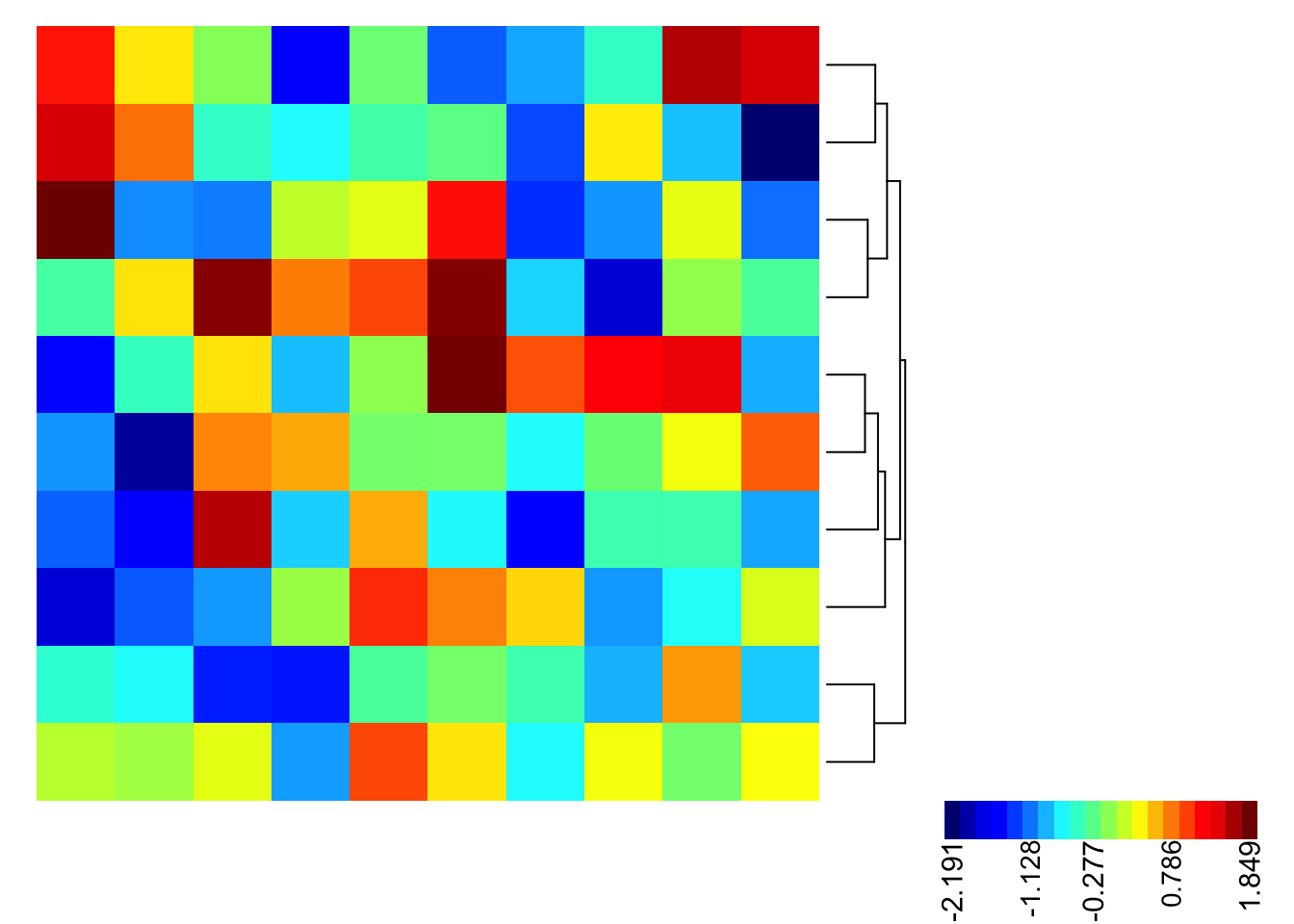Note that if we use edge anchor to place the legend, the legend will be the same length or height as the anchored object. For example, this is how `RightOf` looks like

``p + WLegendV('a',RightOf(pad=0.05, width=0.05), height=100000, width=9999)``